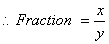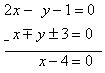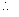# the sum of the numerator and denominator of a fraction is 3 less than twice the denominator. if the numerator and denominator are decreased by 1,the numerator becomes half the denomiator.find the fraction.

let the fraction be x / y

then acc. to ques.

x + y + 3= 2y

x = y - 3 ............. (i)

also

x-1/y-1 = y/2y = 1/2

x - 1 =( y-1) / 2

x = ( (y - 1) / 2 ) +1

x = (y +1) / 2

2x = y + 1

2x - y = 1 ............... (ii)

Subtituting the value of (i) in (ii) we have

2 ( y - 3 ) - y =1

2y - 6 -y = 1

y - 6 = 1

y = 7

x = y - 3

x = 7 - 3

x = 4

Therefore the fraction is x / y = 4 / 7

Thumbs up if you like my answer

• 166

Let the fraction be x/y

According to the question,

x + y + 3 = 2y

x = y - 3  ------(1)

Also, x-1/y-1 = y/2y = 1/2

x - 1 = (y-1) / 2

x = [ (y - 1) / 2 ] +1

x = (y +1) / 2

2x = y + 1

2x - y = 1 ------ (2)

Subtituting the value of (1) in (2), we get:

2 ( y - 3 ) - y =1

2y - 6 -y = 1

y - 6 = 1

y = 7

x = y - 3

x = 7 - 3

x = 4

Therefore the fraction is x / y = 4 / 7

Please Gimme• 16

Let numerator be x and denominator be yA/Q x + y = 2y - 3

or, x - y + 3 = 0 -------------(1)

and x - 1 = 1/2(y - 1)

or, 2x - y - 1 = 0 ---- -----(2)

Subtracting equation (1) form (2) we getx = 4

From (1) 4 - y + 3 = 0

y = 7Fraction = x/y = 4/7

• 15

how do blue green algea and symbiotic bacteria convert atmospheric nitrogen into nitrogen compounds

• -14

-Let numerator be x and denominator be y

A/Q x + y = 2y - 3

or, x - y + 3 = 0 -------------(1)

and x - 1 = 1/2(y - 1)

or, 2x - y - 1 = 0 ---- -----(2)

Subtracting equation (1) form (2) we get

x = 4

From (1) 4 - y + 3 = 0

y = 7

Fraction = x/y = 4/7

• 8
the fraction is 4/7
• -5
1+ 1=2 1+√_1^2 1+√1 1+√1. [ Root of 1 is 1 ] 2
• -3
• -4

let the fraction be x / y

then acc. to ques.

x + y + 3= 2y

x = y - 3 ............. (i)

also

x-1/y-1 = y/2y = 1/2

x - 1 =( y-1) / 2

x = ( (y - 1) / 2 ) +1

x = (y +1) / 2

2x = y + 1

2x - y = 1 ............... (ii)

Subtituting the value of (i) in (ii) we have

2 ( y - 3 ) - y =1

2y - 6 -y = 1

y - 6 = 1

y = 7

x = y - 3

x = 7 - 3

x = 4

Therefore the fraction is x / y = 4 / 7

Thumbs up if you like my answer

• 0
What are you looking for?